# Riesz decomposition property

Let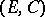be a partially ordered vector space, [a5], i.e.is a real vector space with a convex conedefining the partial order by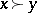if and only if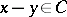. For, the corresponding interval is.
The (partially) ordered vector spacehas the Riesz decomposition property iffor all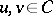, or, equivalently, if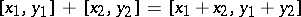for all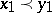,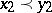.
There is a natural non-commutative generalization to the setting of-algebras, as follows, [a4]. Let,,be elements of a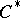-algebra. If, then there aresuch that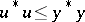,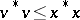and.